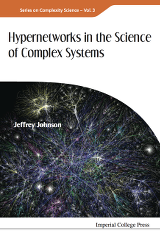Visit Publisher

This book aims to persuade you that the theory of hypernetworks can be useful in science and its applications to complex systems. You probably already know quite a lot about hypernetworks. Networks are one-dimensional hypernetworks, and hypergraphs are precursors to hypernetworks. The starting point is the realisation that networks, excellent though they are for representing relationships between pairs of things, cannot represent relationships between three, four, five or higher numbers of things.

Certainly n-ary relations are common, perhaps more common than binary relations. The need for a formalism to represent n-ary relations has been long known, and hypergraphs have been used for many years for this purpose, along with the beautiful Galois lattice structure they possess. Bipartite relations generate two hypergraphs.

If $$R$$ is a bipartite relation between sets $$A$$ and $$B$$, for each $$a$$ in $$A$$ let $$R(b)$$ be the subset of $$B$$ with elements related to $$A$$. This class of subsets forms a hypergraph. With all its intersections it forms what is here called a Galois hypergraph. There is another hypergraph for the subsets of $$A$$ related to the elements of $$B$$, and with all its intersections it too forms a Galois hypergraph. There is then a Galois connection between the two Galois hypergraphs with the usual Galois lattice. This is very elegant and has been known for many years.

The problem with hypergraphs is that they set-theoretic structures. By definition an edge in a hypergraph is a set of vertices. Because the elements of sets are not ordered, in general $$\{a, b \} = \{ b, a \}$$ and there is ambiguity. Of course in practice this ambiguity is overcome by imposing an order on the vertices, but then the hyper-edge becomes more than a set. In algebraic topology, an ordered set of vertices is a simplex. Thus n-ary relations can be modelled by simplicial complexes. The simplicial complexes of relations have similar Galois lattice properties to hypergraphs.

In the nineteen sixties the English mathematician R. H. Atkin suggested that simplicial complexes could be used to model relations in social systems. An abstract $$p$$-simplex is an ordered list of abstract objects called vertices, $$\sigma_p = \langle v_0, v_1, ... , v_{p_i}\rangle$$. Such abstract simplices can be given a geometric realisation as a $$p$$-dimensional polyhedron. Thus $$\langle v_0 \rangle$$ is identified with a 0-dimensional vertices, $$\langle v_0, v_1 \rangle$$ a 1-dimensional edge, $$\langle v_0, v_1, v_2 \rangle$$ a 2-dimensional triangle, $$\langle v_0, v_1, v_2, v_3 \rangle$$ a 3-dimensional tetrahedron, and so on. After experimenting with the homological structure of relations Atkin suggested a new kind of connectivity based on the shared faces of simplices . For example, if two tetrahedral share a triangular face they are 2-near, or $$q$$-near for $$q$$ = 2. The transitive closure of the $$q$$-nearness relation, $$q$$-connectivity, is an equivalence relation on a set of simplices and partitions them into $$q$$-connected components . A listing of these components for each $$q$$ value is called a $$Q$$-analysis. Atkin also introduced new way of thinking about the topological structure of complexes through his theory of pseudo-homotopy, or $$Q$$-shomotopy. The great advantage of $$Q$$-analysis over as analytic methods was that it allowed the data to "speak for themself" since the shared face $$q$$-nearness can be inspected directly.

Atkin developed his theory of $$Q$$-analysis to include the notion of backcloth and traffic, where the backcloth of relatively static simplicial complexes supports a traffic relatively dynamic patterns of mappings. An important idea is that, as in space-time physics, the dynamics of the traffic are constrained by the geometry and topology of the backcloth. Atkin developed his theory through many applications and these are documented in his many books and papers.

Although a big step forward from hypergraphs, simplicial complexes do not explicitly model the relations that define simplices. This book suggests a new structure, the hypersimplex, in which the relation is explicit, e.g. $$\langle v_0, ... , v_p; R_i \rangle$$. This resolves the problem of discriminating the same set of vertices being related differently, e.g. $$\langle d, g, o; R_{\rm{god}}\rangle \neq \langle d, g, o; R_{\rm{dog}}\rangle$$ while $$\{ g, o, d \} = \{ d,o,g \}$$ and $$\langle g,o,d \rangle = \langle d,o,g\rangle$$. Hypernetworks are collections of hypersimplices. These "relational simplices" or "hypersimplices" open up new possibilities, including relational algebra, e.g. $$\langle v_0,..., v_p; R_1 \star R_2 \rangle$$ and more generally $$\langle v_0, ... , v_p; R_0 \star R_1 \star ... \star R_q\rangle$$, as needed for multiplex structures with many heterogeneous relations combined in many heterogeneous ways.

Hypersimplices provide a way of moving between different levels of assembly and are the basis of a new theory of aggregation and disaggregation in multilevel systems with the objective of unifying the bottom-up, top-down, and middle-out backcloth-traffic dynamics of complex systems at all micro-, meso- and macro-levels. This is the ultimate aim of the research. This book develops these technical ideas in the context of many applications. Many of the initial ideas will be familiar to most readers but it is hoped that all will find something new and useful in the theory of multilevel hypernetworks. The book concludes with a discussion of the design of complex systems, including the design of complex multilevel social systems in the context of policy. The argument is that complex systems science, design and policy are inextricably entangled.

This book reports work in progress. No doubt it contains misconceptions and errors, but the thrust is that hypernetworks are necessary, if not sufficient, for an applicable science of complex systems. Anyone who wants to join in this research and take it forward with constructive criticism and new ideas will be very welcome.